## You pull straight up on the string of a yo-yo with a force 0.35 N, and while your hand is moving up a distance 0.16 m, the yo-yo moves down

Question

You pull straight up on the string of a yo-yo with a force 0.35 N, and while your hand is moving up a distance 0.16 m, the yo-yo moves down a distance 0.32 m. The mass of the yo-yo is 0.062 kg, and it was initially moving downward with speed 2.9 m/s.(a) What is the increase in the translational kinetic energy of the yo-yo?(b) What is the new speed of the yo-yo?(c) What is the increase in the rotational kinetic energy of the yo-yo?

in progress 0
7 months 2021-07-14T06:58:57+00:00 2 Answers 9 views 0

## Answers ( )

a) 0.138J

b) 3.58m/S

c) (1.52J)(I)

Explanation:

a) to find the increase in the translational kinetic energy you can use the relation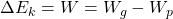where Wp is the work done by the person and Wg is the work done by the gravitational force

By replacing Wp=Fh1 and Wg=mgh2, being h1 the distance of the motion of the hand and h2 the distance of the yo-yo, m is the mass of the yo-yo, then you obtain: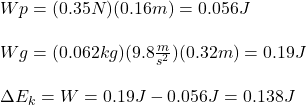the change in the translational kinetic energy is 0.138J

b) the new speed of the yo-yo is obtained by using the previous result and the formula for the kinetic energy of an object: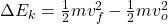where vf is the final speed, vo is the initial speed. By doing vf the subject of the formula and replacing you get: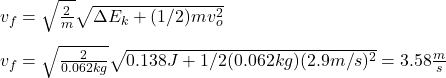the new speed is 3.58m/s

c) in this case what you can compute is the quotient between the initial rotational energy and the final rotational energy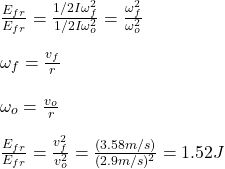hence, the change in Er is about 1.52J times the initial rotational energy

2. Given Information:

Force = F = 0.35 N

distance of hand = h = 0.16 m

distance of yo-yo = y = 0.32 m

mass of yo-yo = 0.062 kg

initial velocity = vi = 2.9 m/s

Required Information:

a) Translational kinetic energy = ΔTKE = ?

b) Final velocity = vf = ?

c) Rotational kinetic energy = ΔRKE = ?

a) Translational kinetic energy = ΔTKE = 0.0413 Joules

b) Final velocity = Vf = 3.12 m/s

c) Rotational kinetic energy = ΔRKE = 0.251 Joules

Explanation:

a) The change in translational kinetic energy of the yo-yo is given by

ΔTKE = (mg – F)h

Where F is the force exerted by the hand on the yo-yo and h is the distance your hand has moved.

ΔTKE = (0.062*9.81 – 0.35)*0.16

ΔTKE = 0.0413 Joules

b) The new speed of the yo-yo can be found using

ΔTKE = ½mVf² – ½mVi²

Re-arranging the equation for the final velocity of the yo-yo,

½mVf² = ΔTKE + ½mVi²

½*0.062*Vf² = 0.0413 + ½*0.062*(2.9)²

0.031*Vf² = 0.0413 + 0.261

Vf² = 0.3023/0.031

Vf² = 9.75

Vf = √9.75

Vf = 3.12 m/s

c) The increase in the rotational kinetic energy of the yo-yo can be found using

ΔTKE + ΔRKE = mgh + Fd

ΔRKE = mgh + Fd + ΔTKE

ΔRKE = 0.062*9.81*0.16 + 0.35*0.32 + 0.0413

ΔRKE = 0.0973 + 0.112 + 0.0413

ΔRKE = 0.251 Joules